Next: Stability of the SD S Up: Superdeformed bands in S Previous: Dynamical moments and relative

# Superdeformed bands in one-particle and one-hole neighbors of 32S

In order to analyze polarization effects induced by individual particle or hole orbitals in 32S, we have also performed the HF calculations for the four neighboring nuclei: 33S, 31S, 33Cl, and 31P. Among them, there are two pairs of mirror nuclei, 33S - 33Cl and 31S - 31P. For each of these nuclei we have calculated four bands, corresponding to either the four lowest available particle states (in A=33), or the four highest available hole states (in A=31). In other words, in 33S or 33Cl the neutron or proton is added to the magic 323232S configuration, in the 3/2(r=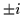) and 5/2(r=) orbitals, which gives the neutron or proton configurations: 32+, 32-, 33, and 33*. Here, by an asterisk we denote the configuration in which a particle is added not to the lowest available intruder state, but to the next-to-lowest available intruder state. Similarly, in 31S and 31P the neutron or proton is removed from the magic 3232 32S configuration, from the 1/2(r=) and 1/2(r=) orbitals, which gives the neutron or proton configurations: 32+, 32-, 31, and 31*. Again, by an asterisk we denote the configuration in which a particle remains not in the lowest available intruder state, but in the next-to-lowest available intruder state.

In Figs. 11 and 12 we show energies of the calculated HF bands in 33S, 31S, 33Cl, and 31P. One can observe that the mirror nuclei have extremely similar SD spectra. Bands in the A=33 nuclei form pairs of degenerate signature partners, while those corresponding to the signature partners in A=31 are strongly split, in accordance with the characteristic features of the corresponding single-particle routhians, Figs. 1 and 2. Note that the ground state bands in the A=33 nuclei correspond to non-intruder particle states, and similarly, those in the A=31 nuclei correspond to holes in non-intruder orbitals.

The HF calculations give the energies of rotational bands on the absolute scale. Therefore, in order to estimate the available Q-value windows for particle emissions, one may simply compare (at a given value of the angular momentum) the energies shown in Figs. 6, 11, and 12. Since the rigid-rotor reference energies are the same at fixed spins, one can directly compare the values given in the figures. For example, for 31S the yrast energy at I=12 is about -244MeV, which shows that none of the 32S bands shown in Fig. 6, except the 3434 and 3034configurations, can emit a zero-angular-momentum neutron to the SD states in 31S. Similarly, for 31P the corresponding yrast energy is -249.5MeV, which opens up the proton emission channel from several other bands, but not those from the near-yrast bands shown in Fig. 7.

Let us emphasize that the angular momentum,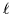, carried away by an emitted particle, dramatically influences the considered Q-values in nuclei around 32S, especially at high spins. Since after subtracting the rigid-rotor reference, the energies of bands are fairly flat (Figs. 6, 11, and 12), one can very simply estimate the Q-values at given I andvalues to be by an amount of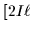+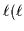+1)]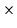0.05MeV larger than those at=0. For instance, at I=20, and with the angular-momentum transfer=2 (or 3), the additional energies in a daughter nucleus are 4.3 (or 6.6)MeV. Consequently, the protons emitted through the high angular-momentum (e.g., N0=3) orbitals are among the most likely candidates for the band-to-band emission mechanism. From the results presented in the figures one may precisely estimate the Q-value windows for particles carrying out any given amount of the angular momentum from any given band.

The illustrations of the dynamical moments in 33S, 31S, 33Cl, and 31P, shown in Figs. 13 and 14, indicate an extreme similarity of the results in mirror nuclei. This suggests that several among the SD bands in the mirror nuclei around 32S might manifest the identical band'' phenomenon. Comparing these results with those in the magic SD band in 32S, Fig. 9, one sees that particles in the intruder 3/2 orbitals and extruder 5/2 orbitals, respectively add and subtract 1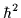/MeV(at high spin) with respect to the magic core. Variations of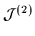, that correspond to the intruder and non-intruder hole states, are of the similar order.

By calculating differences between one-body observables, like the angular momentum or quadrupole moment, determined in 33S, 31S, 33Cl, and 31P, and in 32S, one can identify basic single-particle properties of all important orbitals around the SD 32S magic-core configuration. These differences correspond not only to the bare average values of the observables, calculated for given orbitals, but also include complete polarization effects. It is known that in the SD A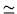150 nuclei, the single-particle alignments , and charge quadrupole moments [43,44], constitute additive quantities with respect to adding and subtracting particles from the magic SD configurations of 152Dy. An analogous observation is also confirmed by calculations in the SD A60 nuclei [29,45,46]. In the present paper we have verified the additivity of alignments and quadrupole moments between the SD bands in 32S, and in 33S, 31S, 33Cl, and 31P. Tests of this principle in other nuclei around 32S are left for a future publication.

In Figs. 15 and 16 we present the obtained relative alignments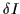and proton quadrupole moments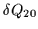, respectively. Since the relative alignments pertain to the total angular momentum, the effects of neutron and proton orbitals, obtained in the N=16 and Z=16 nuclei, respectively, are almost identical. For relative proton quadrupole moments, the effects of neutrons and protons are different, because neutrons contribute only through the polarization effects, while for protons one also has the bare direct contribution. In Figs. 15 and 16 we also indicate by which particle- or hole-orbital differ the bands in 33S, 31S, 33Cl, and 31P form the magic SD band in 32S.

One can see that the relative alignments generated by various orbitals differ considerably. Therefore, the relative alignments may serve as distinct fingerprints of orbitals in SD nuclei around 32S. In particular, the second intruder, hole-orbital 1/2(r=-i), gives rather large negative relative alignment, while the positive-parity, hole-orbital 1/2(r=+i), gives a rather constant alignment of about -1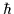, and hence may be at the origin of hypothetical yet another class of identical bands in this region.

The relative proton quadrupole moments of orbitals around the magic N=Z=16 SD gap are fairly constant in function of the rotational frequency. Values corresponding to intruder orbitals are usually much larger than those corresponding to positive-parity orbitals. Hence, one can easily understand the origin of groups of 32S bands having significantly different quadrupole moments, see Sec. 4.2. As far as the polarization effects alone are concerned, the extruder particle orbitals 5/2(r=) carry almost the same effect as the intruder hole-orbitals 1/2(r=). Needless to say, these are the main orbitals which are at the origin of the SD shapes in 32S.Next: Stability of the SD S Up: Superdeformed bands in S Previous: Dynamical moments and relative
Jacek Dobaczewski
1999-07-27